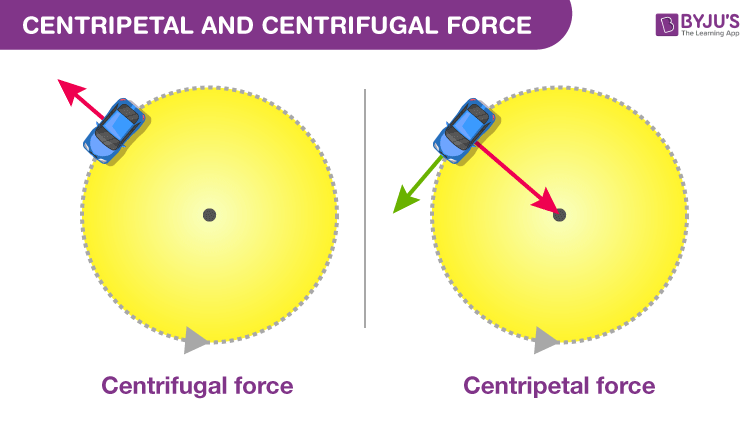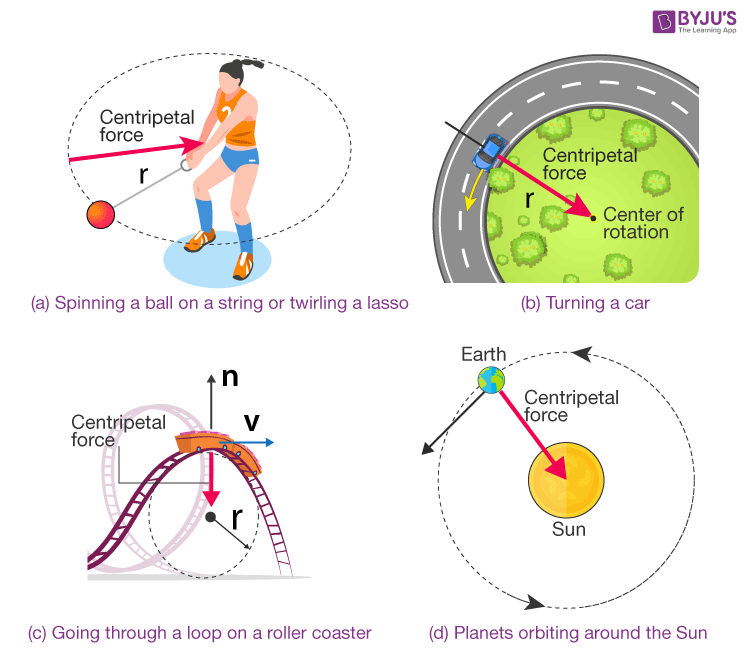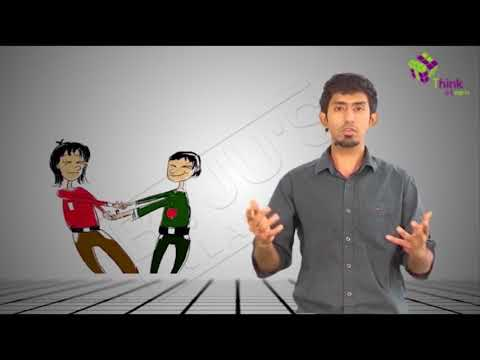# Centripetal And Centrifugal Force

To make an object move, force is required. Force acts differently on objects depending on the type of motion it exhibits. In the case of curvilinear motion, two types of force come into the picture, i.e., the centrifugal force and centripetal force. Centripetal force is the force acting towards the centre of the circular path. Whereas, the centrifugal force acts in a direction pointing away from the centre of the circle. In this article, let us discuss centripetal and centrifugal force in detail.## What is Centripetal Force?

Centripetal force is defined as,

The component of force acting on an object in curvilinear motion which is directed toward the axis of rotation or center of curvature.

The unit of centripetal force is Newton. The centripetal force is always directed perpendicular to the direction of the displacement of the object.

If an object accelerates according to the changes of velocity, then it can change either its speed or direction of motion. In simple terms, if any moving object in a circular path is constantly changing its direction means it is constantly accelerating. Using Newton’s second law of motion, if an object is travelling in a circular path, it is found that the centripetal force of an object moving in a circular path always acts towards the centre of the circle.

### How is the Centripetal Force Calculated?

The Centripetal Force Formula is given as the product of mass (in kg) and tangential velocity (in meters per second) squared, divided by the radius (in meters). Which implies that on doubling the tangential velocity, the centripetal force will be quadrupled. Mathematically it is written as:

 $F=ma_{c}=\frac{mv^{2}}{r}$

Where,

• F is the Centripetal force.
• ac is the Centripetal acceleration.
• m is the mass of the object.
• v is the speed or velocity of the object.

### Centripetal Force Examples

The force that pulls or pushes an object toward the centre of a circle as it travels, causing angular or circular motion is called a Centripetal Force. Some examples of Centripetal Force is given below.A few examples of Centripetal Force

• Spinning a ball on a string or twirling a lasso: Here the centripetal force is provided by the force of tension on the rope pulls the object in toward the centre.
• Turning a car: Here the centripetal force is provided by the frictional force between the ground and the wheels.
• Going through a loop on a roller coaster: The force is provided by the Normal Force as the seat or wall pushes you toward the centre.
• Planets orbiting around the Sun: Centripetal Force is provided by Gravity.
 Related Links on Centripetal Force Centripetal Acceleration Radial Acceleration Derivation of Centripetal Acceleration Central Force

## What is Centrifugal Force?

If an object moving in a circle and experiences an outward force than this force is called the centrifugal force. However, the force also depends on the mass of the object, the distance from the centre of the circle and also the speed of rotation. If the object has more mass, the force of the movement and the speed of the object will be greater. If the distance is far from the centre of the circle the force of the movement will be more.

The Centrifugal Force is defined as,

The apparent force, which is equal in magnitude and opposite in direction to the centripetal force, drawing a rotating object away from the center of rotation, caused by the inertia of the object.

Centrifugal force unit is Newton. The centrifugal force drives the object away from the centre. It is a fictitious force.

### How is Centrifugal Force calculated?

Centrifugal Force Formula is given as the negative product of mass (in kg) and tangential velocity (in meters per second) squared, divided by the radius (in meters). Which implies that On doubling the tangential velocity, the centripetal force will be quadrupled. Mathematically it is written as:

 $F_{c}=-\frac{mv^{2}}{r}$

Where,

• Fc is the Centrifugal force
• m is the mass of the object
• v is the velocity or speed of the object.

### Centrifugal Force Examples

Centrifugal Force acts on every object moving in a circular path when viewed from a rotating frame of reference. Some examples of Centrifugal Force are given below.

• Weight of an object at the poles and on the equator
• A bike making a turn.
• Vehicle driving around a curve
• Equatorial railway

## Why does the Moon keep going around the Earth?## Centripetal Force Vs Centrifugal Force

Check the table below to learn the detailed comparison between Centripetal and Centrifugal Force

Differences Between Centripetal And Centrifugal Force
Centrifugal Force
Centripetal Force
If an object moving in a circle and experiences an outward force than this force is called the centrifugal force If the object travels in a uniform speed in a circular path is called centripetal force.
The object has the direction along the centre of the circle from the centre approaching the object The object has the direction along the centre of the circle from the object approaching the centre.
Mud flying of a tire is one example of the centrifugal force. A satellite orbiting a planet is an example of the centripetal force.

## Centripetal and Centrifugal Force FAQs

Q1: What is Centripetal force?
Ans: Centripetal force is defined as the force required to keep a body moving in a circular path and direct towards the centre of a circle.

Q2: Give examples of Centripetal force.
Ans: Following are the examples of centripetal force:

• The revolution of the moon around the earth.
• The spinning of the top.
• Rides in a roller coaster.

Q3: What is the formula of centrifugal force?
Ans: $F_{c}=-\frac{mv^{2}}{r}$ Where,

• Fc is the centrifugal force
• m is the mass
• v is the speed

Q4: What is the formula of centripetal force?
Ans: $F=\frac{mv^{2}}{r}$ Where,

• F is the centripetal force
• m is the mass
• v is the speed

Q5: Compare Centrifugal force and centripetal force.
Ans: Centrifugal force is equal in magnitude and opposite in direction to the centripetal force.

Stay tuned with BYJU’S to learn more interesting physics topics with the help of interactive video lessons.